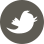# Regional Semantic Contrast and Aggregation for Weakly Supervised Semantic Segmentation 笔记

8月 28, 2022 - 算法

## 引言

• 语义对比，这种方式让模型学习区分数据集中所有可能的对象区域，从而促进更全面的对象模式理解。具体来说，对于每个伪区域，语义对比使得网络将其语义嵌入向记忆库中相同类别的嵌入方式拉近，同时将其向不同的类别推远。这种对比方式为单张图像补充了分类目标，从而改进对象的表示学习。
• 语义聚合，这种方式使得模型可以收集数据集级别的上下文知识以产生更有意义的对象表示。这是方法通过一个没有参数的注意力机制模块实现的，该模块独立地总结了每个图像的记忆表示。与传统的图像内上下文学习方案相比，我们的语义聚合侧重于图像间上下文挖掘，因此能够捕获更多信息的数据集级语义。

1. 我们研究了WSSS中至关重要但是却被长期忽视的问题，以探索用于网络训练的弱标记数据的更丰富的上下文信息。这实质上缩小了图像级语义概念和像素级对象区域之间的差距。
2. 具体来说，我们引入了一个用于语义对比的鲁棒的对比学习算法，该算法可以从不完美的伪区域中学习更为有效的表示。
3. 同时，还有一个非参数化的用于特征聚合的注意力模型，该模型可以从整个数据集中收集丰富的上下文知识。

## 方法

### 问题描述

#### 先前的WSSS解决方案

\mathbf{F} = F_{FCN}(I) \in \mathbf{R}^{W\times H\times D}, \mathbf{P} = F_{CAM}(\mathbf{F}) \in \mathbf{R}^{W\times H\times L}

### 区域语义对比和聚合

#### 伪区域表示

f_l = \frac{\sum_{x=1,y=1}^{W,H} M_l(x,y)\mathbf{F}(x,y)}{\sum_{x=1,y=1}^{W,H} M_l(x,y)} \in \mathbf{R}^D

#### 伪区域记忆库

m_l \leftarrow \gamma m_l + (1-\gamma)f_l

#### 记忆机制的讨论

1. 记忆被划分得足以单独压缩每个训练样本中的每个潜在语义假设，并且能够在弱标记的视觉数据中很好地编码每个类别的不同语义模式。
2. 上述动量更新方案不仅可以有助于为语义对比获得一致的记忆特征，而且提供了能够更精确描述目标语义的综合表示。具体来说，这种动量更新方案积累了不同训练epoch中由图像分类器产生的每个对象区域的所有中间状态。这些中间状态之间显示出了很好的互补性，并且每个记忆特征m_l都能随着训练进行逐渐提升，从而捕捉一个更完整的对象区域。这最终会在训练后产生信息丰富的记忆表示，可以将其用作语义聚合的可靠上下文

#### 区域语义对比

\mathcal{L_l^{NCE}} (f_l,y_l)= \frac{1}{|M_l|} \sum_{m_l^+ \in M_l} -log\frac{e^{sim(f_l, m_l^+)/\tau}}{e^{sim(f_l, m_l^+)/\tau} + \sum_{m_l^-\in M \setminus M_l }e^{sim(f_l,m_l^-)/\tau}}

\hat{f_l} = \omega f_l + (1 – \omega)f_{l^-}

\mathcal{L_{l}^{RM-NCE}} = \omega \mathcal{L_l^{NCE}}(\hat{f_l},y_l) + (1 – \omega)\mathcal{L_l^{NCE}}(\hat{f_l}, y_{l^-})

• 根据类别得到语义嵌入f_l，之后开始混合操作
• 从mini-batch中随机选择一张图像的其中一个类别l^-，根据相同的语义嵌入方法得到f_{l^-}
• 之后根据\omega混合嵌入公式将其线性结合，得到\hat{f_l}
• 取出整个记忆库，大小为((L\times lens), D)，分别对记忆库M和f_l进行l_2标准化
• 利用torch.einsum()计算\hat{f_l}和记忆库中每一项的余弦相似度，大小为(L\times lens)的向量
• 将该向量与温度\tau相除，得到sim(f_l, m_l)/\tau，记其中最大值为max_log
• 将向量减去max_log，再加上exp操作，得到exp_logit
• 计算正样本时，与上述计算方式类似，只是将全部的m_l替换成与当前类别对应的m_l^+
• 计算负样本时，先生成一个长度为(L\times lens)的二元向量，其中与当前类别对应的L长度部分的值为False，其余位置为True，将其与exp_logit相乘后再求和，相当于消除正样本的影响
• 之后根据NCE公式计算损失函数。
• 这样的操作针对当前类别ll^-分别进行，依据参数\omega混合求得的损失，之后在总Loss上加上该项与当前图像总类别数倒数的乘积。

#### 区域语义聚合

S = softmax(F\otimes Q^T) \in \mathbf{R^{(WH)\times(LK)}}

F’ = S \otimes Q \in \mathbf{R}^{(WH)\times D}

\hat{F} = [F, F’] \in \mathbf{R}^{W\times H \times 2D}

#### CAM预测

O = F_{CAM}(\hat{F}) \in \mathbf{R}^{W\times H\times L}

### 详细网络结构

1. backbone网络F_{FCN}，将输入图像I转换为一个卷积表示F。任何FCN网络都可以使用，我们使用了VGG16和ResNet38.

2. 类别级卷积层F_{CAM}从特征嵌入中产生类感知attention map。在我们的网络中，产生粗略map的和最终CAM预测的是不同的F_{CAM}

3. 记忆库M存储所有训练过程中产生的区域模式，在inference阶段，记忆库被移除，仅有K-means产生的原型表示保留下来。这降低了在模型部署期间维护大型记忆库的成本。

4. 损失函数为：
\mathcal{L} = \sum_I\alpha_1 \mathcal{L}^{RM-NCE}+\alpha_2\mathcal{L}^{CE}(GAP(P), y) + \mathcal{L}^{CE}(GAP(O), y)
其中每张图像I都被3个损失项监督。第一项\mathcal{L}^{RM-NCE}是区域混合对比损失，其值为图像I中所有区域的平均值。第二项是辅助交叉熵损失\mathcal{L}^{CE}，用于监督中间产生的CAM预测P，第三项则是用于监督最终产生的CAM预测O